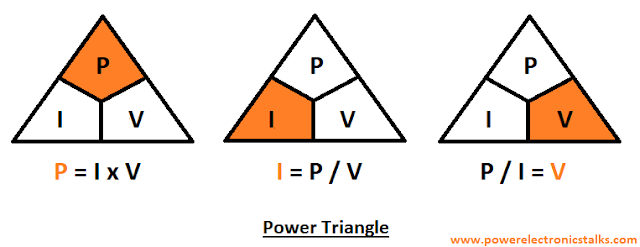# Ohm's law Calculations and Calculator

Ohm’s law many of us heard many times. To understand ohm’s law is very important for electrical and electronics engineer, technicians, hobbyist, etc. Here we will discuss in detail about Ohm’s law definition, Ohm’s law calculations and calculator. Also, a Ohms law calculator excel sheet also provided.Ohm's Law

### Ohm’s law Calculator

Enter any two known values and press "Calculate" to get solutions of the others.
Fields should be reset to 0 or clear before each new calculation.
Volts
Amps
Ohms
Watts

This Ohm’s law calculator will help you to perform ohm’s law calculations easily. Even manually calculated values using ohm’s law formula you can compare with the results of this calculator.

### Ohms law Calculator Excel Sheet

An excel sheet for Ohm's law calculation you can access by clicking on below button. Download it and save in your laptop, computer, tablet or mobile;Ohm's law Calculator
Now let discuss Ohm’s law definition and its calculation in detail;

### What is Ohm’s law

Definition of Ohm’s law is, “the current through a conductor between two points is directly proportional to the voltage across the two points”.

Introduce Resistance as constant of proportionality. Resistance in below equation is constant and not dependent of the current.

The German physicist Georg Ohm invented this law. As per him, at a constant temperature, the current flowing through a fixed linear resistance is directly proportional to the voltage applied across it, and inversely proportional to the resistance.

In other words, we can say that, the relationship between Voltage, Current and Resistance is called as Ohm’s law.

Ohm's law is an empirical relation i.e. experimental relation which precisely defines the conductivity of the many of electrically conductive materials over different level of current.

Non-ohmic material do not follow Ohm's law.

### Ohm’s law equation

The mathematical equation for the same can be given as; Resistance = Voltage / Current.
Where,
Current (I) = current through the conductor in the units of amperes.
Voltage (V) = voltage measured across the conductor in the units of volts.
Resistance (R) = resistance of the conductor in the units of ohms.

### Ohm’s law formula

For calculating voltage, V
V = I x R

For calculating voltage, I
I = V / R

For calculating voltage, R
R = V / I

### Ohms law triangle

We can remember the ohm’s law formula's by the help of picture, which is called as Ohms law triangle.

The voltage, current and resistance in this triangle occupies different spaces and are fixed in these spaces i.e. no variation of spaces is allowed.

In triangle initial position starts with Voltage which is at top. Current and Resistance is at below.

The voltage, current and resistance positions are fixed in the triangle as per Ohm’s law.

Check below triangles to understand Ohm’s law in easy way;Ohm's Law Triangle

### Electric Power and Ohm’s law

We can define electric power as, it is the rate per unit time, an electric circuit transfers electrical energy. Watt one joule per second is the SI unit of power.

It is represented by the letter P.
In terms of voltage and current the Power can be given as; P = V x I

### Electric Power formula using ohm’s law

Also using ohm’s law electric power formula, we can write as;

P = V² ÷ R

P = I² x R

### Power Triangle

We can remember the formula for power by the help of picture, which is called as power triangle.

The power, current and volateg in this triangle occupies different spaces and their positions are fixed in these spaces.

In triangle initial position starts with Power which is at top. Current and Voltage is at below.

Check below triangles to understand electrical power formula's in easy way;Power Triangle

### Ohm’s law wheel

Now we know very well all the formulas related to ohm’s law formula and electric power formula.

By using these formulas, we can design ohms law wheel. According to Voltage, Current, Power, and Resistance twelve different formulas are mentioned in ohms law wheel.

Also, on the basis of same we can create Ohm's law Table of Formulas;

### Conclusion

The person who mostly deals with electrical and electronics terms or mostly with resistance the Ohm's law is an important rule to solve the current, voltage, resistance and power issues. The calculations presented here surely will help to calculate Ohm's law manually and to understand the Ohm's law concept. The calculator presented here will ease the calculation process.

It is somewhat fantastic, and yet check out the advice at this treat. 64 inches in feet